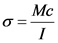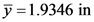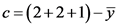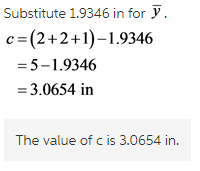# In the bending equation sigma=Mc/I, what is c?

In the bending equation sigma=Mc/I, what is c?

I understand everything about this problem except for the very last step. What is c and how do I find it?

Concepts and reason
Bending characterizes the behavior of a slender structural element like a beam subjected to an external load applied perpendicularly to a longitudinal axis of the element.
Compressive and tensile forces are developed in the direction of the beam axis under bending loads. These forces induce stresses on the beam. When a beam of uniform cross section undergoes sagging moment, the maximum compressive stress occurs at its uppermost edge while the maximum tensile stress occurs at its lower edge. Since the stresses between these two opposing maxima vary linearly, there exists a point on the linear path between them where there is no bending stress. The locus of these points along the length of the beam is the neutral axis.
Uniform cross section beams in bending is not particularly an effective means of supporting a load as it does not use the full capacity of the beam until it is on the verge of collapsing.

Fundamentals
The formula for calculating the bending stress in a beam under simple bending is:Here, the moment about the neutral axis is M, the perpendicular distance from the outermost fiber to the neutral axis is c, and the moment of inertia is I.

Consider the cross section of the beam,

Explanation:
Draw the given figure representing the location of neutral axis from the bottom of the beam and also the point c.

Write the location of the neutral axis from the bottom of the beam (given in the question).Calculate the value of c.Here, the perpendicular distance from the outermost fiber to the neutral axis is c.Explanation:
After calculating the location of the neutral axis from the bottom of the beam, the value of c can be calculated by subtracting location of the neutral axis from the total height of the beam. Here, the value of c is the maximum distance from the neutral axis to the outermost fiber of the beam. If the location of the neutral axis is calculated from the top of the beam, then it equals to c instead.